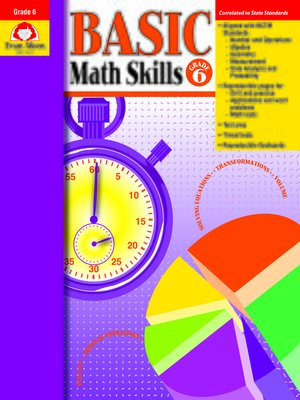We want your feedback!# Basic Math Skills, Grade 6

### Basic Math Skills

#### byEvan-Moor Educational Publishers

ebook

With an OverDrive account, you can save your favorite libraries for at-a-glance information about availability. Find out more about OverDrive accounts.

### Subjects

In Basic Math Skills, Grade 6+, you'll find just what you need to provide extra skill practice for individual students, small groups, or the whole class. These reproducible pages are perfect for homework too. Skills practiced include: Number and Operations • Computation with whole numbers • Computation with fractions • Computation with decimals • Percents and their equivalencies • Number theory Algebra • Function tables • Number lines and the coordinate system • Order of operations • Solving equations Geometry • Identify congruent shapes using transformational geometry • Identify lines of symmetry in two-dimensional shapes • Classify angles as acute, right, or obtuse • Identify characteristics of three-dimensional solids Measurement • Customary and metric measurement • Calculate elapsed time • Measure angles using a protractor • Perimeter, area, and volume Data Analysis and Probability • Construct and interpret graphs • Analyze data using range, mean, median, and mode • Compute theoretical probabilities for simple chance events • Utilize counting techniques, tree charts, and organized lists to determine all possible combinations

### Publication Details

Publisher:
Evan-Moor Corporation
Imprint:
Evan Moor Educational Publishers
Edition:
Teacher's Edition
Publication Date:
2003
Series:
Basic Math Skills